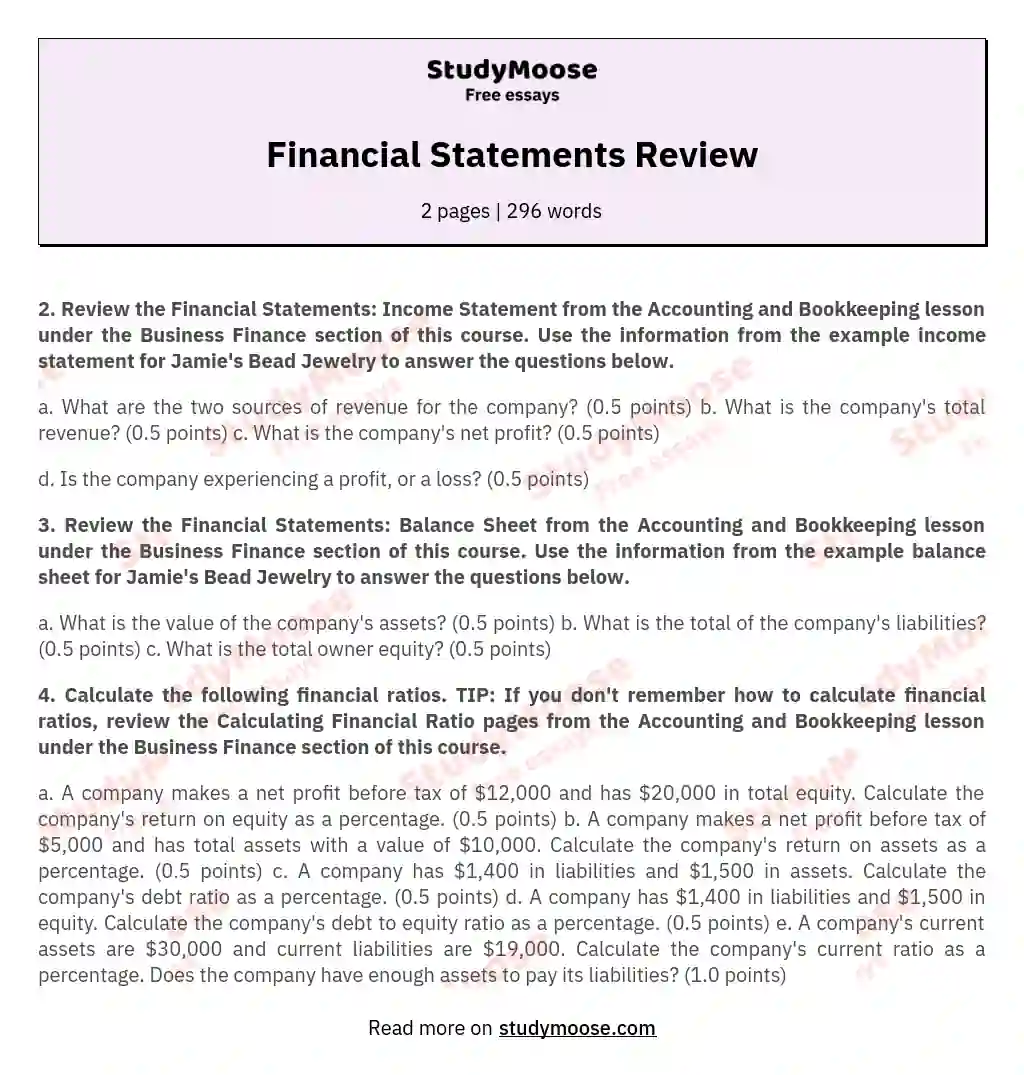# Financial Statements Review

Categories: Taxation

2. Review the Financial Statements: Income Statement from the Accounting and Bookkeeping lesson under the Business Finance section of this course.

Get quality help nowwriter-CharlotteVerified writer

Proficient in: Taxation4.7 (348)

“ Amazing as always, gave her a week to finish a big assignment and came through way ahead of time. ”+84 relevant experts are online

Use the information from the example income statement for Jamie’s Bead Jewelry to answer the questions below.

a. What are the two sources of revenue for the company? (0.5 points)
b. What is the company’s total revenue? (0.5 points)
c. What is the company’s net profit? (0.5 points)

d. Is the company experiencing a profit, or a loss? (0.5 points)

3. Review the Financial Statements: Balance Sheet from the Accounting and Bookkeeping lesson under the Business Finance section of this course. Use the information from the example balance sheet for Jamie’s Bead Jewelry to answer the questions below.

a. What is the value of the company’s assets? (0.5 points)
b. What is the total of the company’s liabilities? (0.5 points)
c. What is the total owner equity? (0.5 points)

4. Calculate the following financial ratios. TIP: If you don’t remember how to calculate financial ratios, review the Calculating Financial Ratio pages from the Accounting and Bookkeeping lesson under the Business Finance section of this course.

a. A company makes a net profit before tax of \$12,000 and has \$20,000 in total equity. Calculate the company’s return on equity as a percentage.

Get to Know The Price Estimate For Your Paper
Topic
Number of pages
Email Invalid email

You won’t be charged yet!

(0.5 points)
b. A company makes a net profit before tax of \$5,000 and has total assets with a value of \$10,000. Calculate the company’s return on assets as a percentage. (0.5 points)
c. A company has \$1,400 in liabilities and \$1,500 in assets. Calculate the company’s debt ratio as a percentage. (0.5 points)
d. A company has \$1,400 in liabilities and \$1,500 in equity. Calculate the company’s debt to equity ratio as a percentage. (0.5 points)
e. A company’s current assets are \$30,000 and current liabilities are \$19,000. Calculate the company’s current ratio as a percentage. Does the company have enough assets to pay its liabilities? (1.0 points)